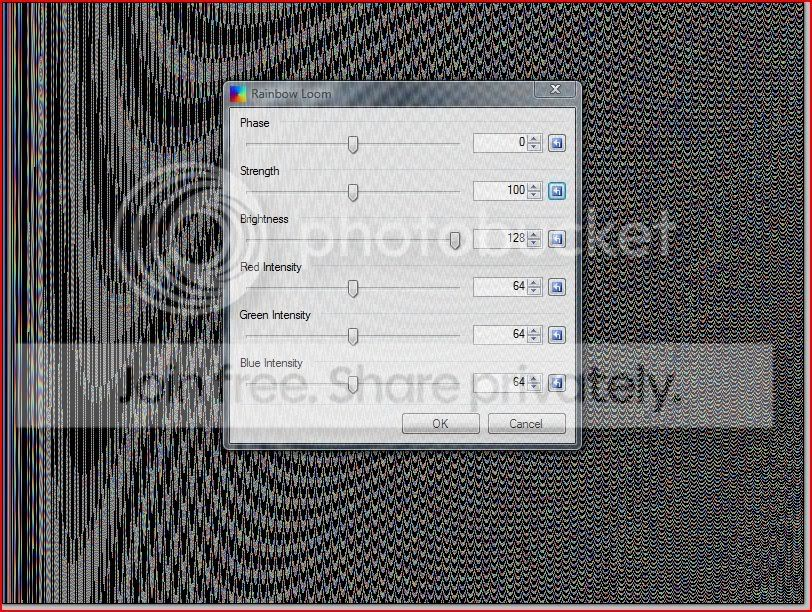Recommended Posts

well, this time I decided to make something a little bit more advanced, so after tweaking some code from Madjik's bubble tut, I was able to come up with this

This plugin renders the loom of your choosing onto the source canvas.(thanks to Ego Eram Reputo for helping me out with the sliders)

enjoy!(this effect is found in render)

rainbow loom.zip

Share on other sites

With all due respect, a plugin that has no configurable parameters is going to be of limited use to a lot of users.

I'd highly recommend a least a couple of sliders to change the pattern and/or orientation.

Share on other sites

Like this:```/* ========================================================================== */
/*                                                                            */
/*   RainbowLoom.cs                                                           */
/*   (c) 2009 person_321 (sliders added by Ego Eram Reputo)                   */
/*                                                                            */
/*   Renders a colorful texture                                               */
/*                                                                            */
/* ========================================================================== */

// Name: Rainbow Loom
// Author: person_321
// URL: http://www.getpaint.net/redirect/plugins.html

#region UICode
int Amount1 = 0; // [-25,25] Phase
int Amount2 = 128; // [0,256] Brightness
int Amount3 = 100; // [10,190] Strength
#endregion

void Render(Surface dst, Surface src, Rectangle rect)
{
int w = dst.Width, h = dst.Height;
for(int y = rect.Top; y     {
for (int x = rect.Left; x         {
int u = x * (y + 2) - 3 / w + (h*x),v = h + w*w*Amount3 *(x*(52+Amount1));
dst[x, y] = Func(Math.Sqrt(u * u + v * v), Math.Atan2(v, u));
}
}
}

ColorBgra Func(double r, double t)
{
r /= 100;
return ColorBgra.FromBgr(
Utility.ClampToByte(Amount2 + 127 * Math.Sin(r + t)),
Utility.ClampToByte(Amount2 + 127 * Math.Sin(Math.PI/2 + r + t)),
Utility.ClampToByte(Amount2 + 127 * Math.Sin(Math.PI + r + t)));

}
```

Share on other sites

Or this:

```/* ========================================================================== */
/*                                                                            */
/*   RainbowLoom.cs                                                           */
/*   (c) 2009 person_321 (sliders added by Ego Eram Reputo)                   */
/*                                                                            */
/*   Renders a colorful texture                                               */
/*                                                                            */
/* ========================================================================== */

// Name: Rainbow Loom
// Author: person_321
// URL: http://www.getpaint.net/redirect/plugins.html

#region UICode
int Amount1 = 0; // [-25,25] Phase
int Amount2 = 100; // [10,190] Strength
int Amount3 = 128; // [0,128] Brightness
int Amount4 = 64; // [0,128] Red Intensity
int Amount5 = 64; // [0,128] Green Intensity
int Amount6 = 64; // [0,128] Blue Intensity
#endregion

void Render(Surface dst, Surface src, Rectangle rect)
{
int w = dst.Width, h = dst.Height;
for(int y = rect.Top; y     {
for (int x = rect.Left; x         {
int u = x * (y + 2) - 3 / w + (h*x),v = h + w*w*Amount2 *(x*(52+Amount1));
dst[x, y] = Func(Math.Sqrt(u * u + v * v), Math.Atan2(v, u));
}
}
}

ColorBgra Func(double r, double t)
{
r /= 100;
return ColorBgra.FromBgr(
Utility.ClampToByte(Amount3 + Amount6 * Math.Sin(r + t)),
Utility.ClampToByte(Amount3 + Amount5 * Math.Sin(Math.PI/2 + r + t)),
Utility.ClampToByte(Amount3 + Amount4 * Math.Sin(Math.PI + r + t)));
}

```

Join the conversation

You can post now and register later. If you have an account, sign in now to post with your account.